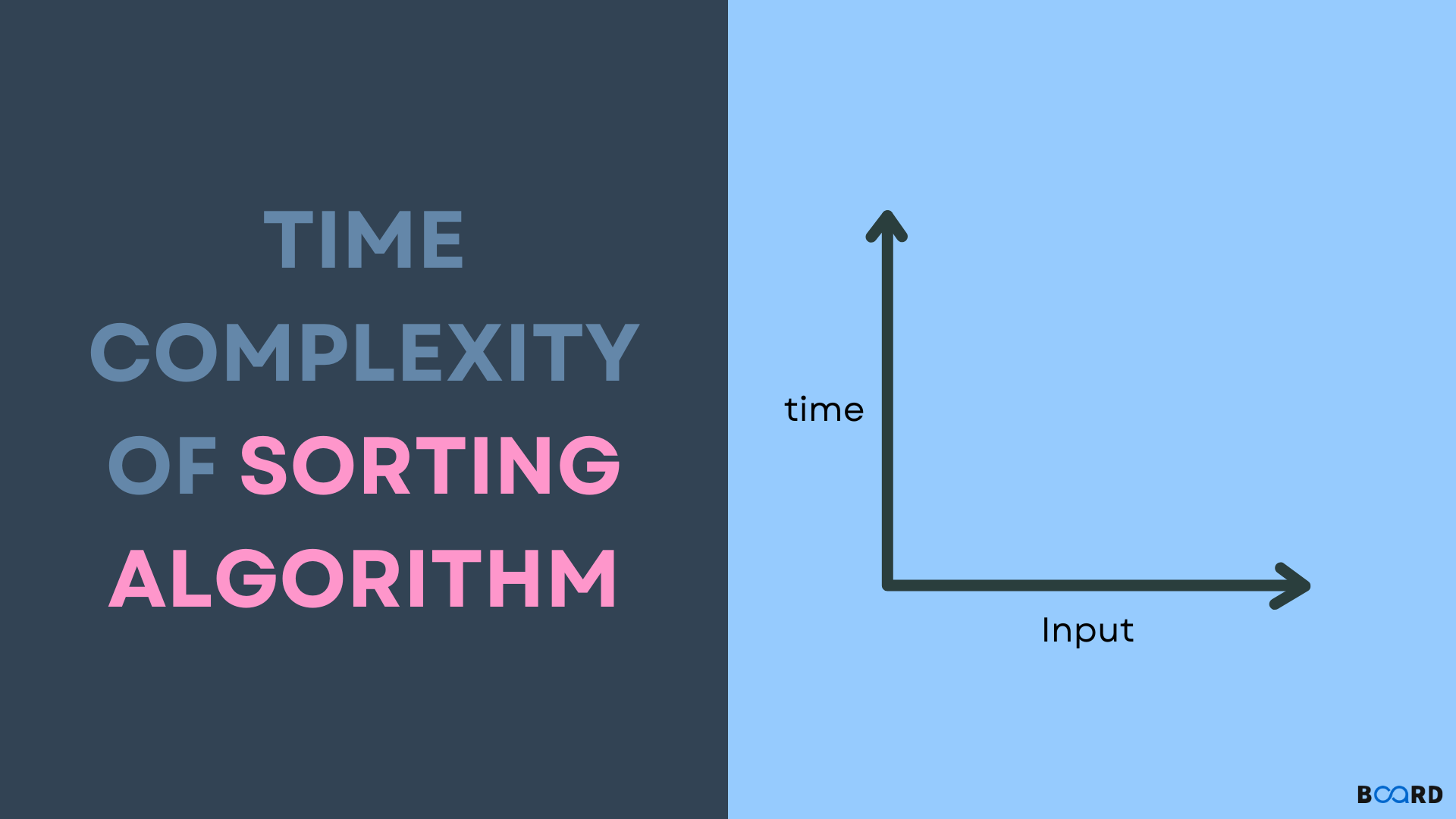# Time Complexity of Sorting Algorithms## Introduction

Whenever we get a complex array, we simply perform sorting. But have you ever thought about what costs to perform a sorting algorithm? At what time complexity is your sorting algorithm working? Don’t worry. We will cover everything in this article.

Time complexity is one of the most important parts of data structure and algorithms. If it is too much then it will cost you so much while doing coding. Your program will going to take lot of time to execute. Time complexity of sorting algorithms is the most asked question in the technical interviews as well. So, be with us until last of this article. We got your back. Before moving on to the time complexity of sorting algorithms, let us understand what time complexity is.

## What is Time Complexity?

An algorithm's time complexity is measured by how many times it must run, depending on the input size. Since elements like the operating system, programming language, and processor capacity are also considered, the time complexity is not a measurement of how long it takes to execute a certain method.

A form of computational complexity known as "time complexity" describes the amount of time needed to carry out an algorithm. The amount of time to complete each statement is the algorithm's time complexity. This basically depends on the volume of the processed data. Time complexity also helps to define the algorithm's performance, and we can also know how efficient the algorithm is to use. Let us discuss what the different types of time complexities are.

## Types of Time Complexity

Time Complexity of Sorting Algorithms

## Time Complexity of Sorting Algorithms

Now we will look at the time complexity of sorting algorithms and discuss all three types of time complexity(Best, Average and Worst) for each algorithm. We will look at merge sort, insertion sort, quick sort time complexity, etc.

 Name of Sorting Algorithm Best Time Complexity Average Time Complexity Worst Time Complexity Insertion Sort Ω(n) θ(n2) O(n2) Bubble Sort Ω(n) θ(n2) O(n2) Merge Sort Ω(n log(n)) θ(n log(n)) O(n log(n)) Quick Sort Ω(n log(n)) θ(n log(n)) O(n2) Selection Sort Ω(n2) θ(n2) O(n2) Tim Sort Ω(n) θ(n log(n)) O(n log (n)) Heap Sort Ω(n log(n)) θ(n log(n)) O(n log(n)) Bucket Sort Ω(n +k) θ(n +k) O(n2) Radix Sort Ω(nk) θ(nk) O(nk) Count Sort Ω(n +k) θ(n +k) O(n +k) Shell Sort Ω(n log(n)) θ(n log(n)) O(n2) Tree Sort Ω(n log(n)) θ(n log(n)) O(n2) Cube Sort Ω(n) θ(n log(n)) O(n log(n))

## Conclusion

In this article, we have discussed about the time complexity of sorting alogortihms. We have also discussed various types of time complexities. Before using a sorting algorithm, you can determine how much time it will take, and then you can use it accordingly.